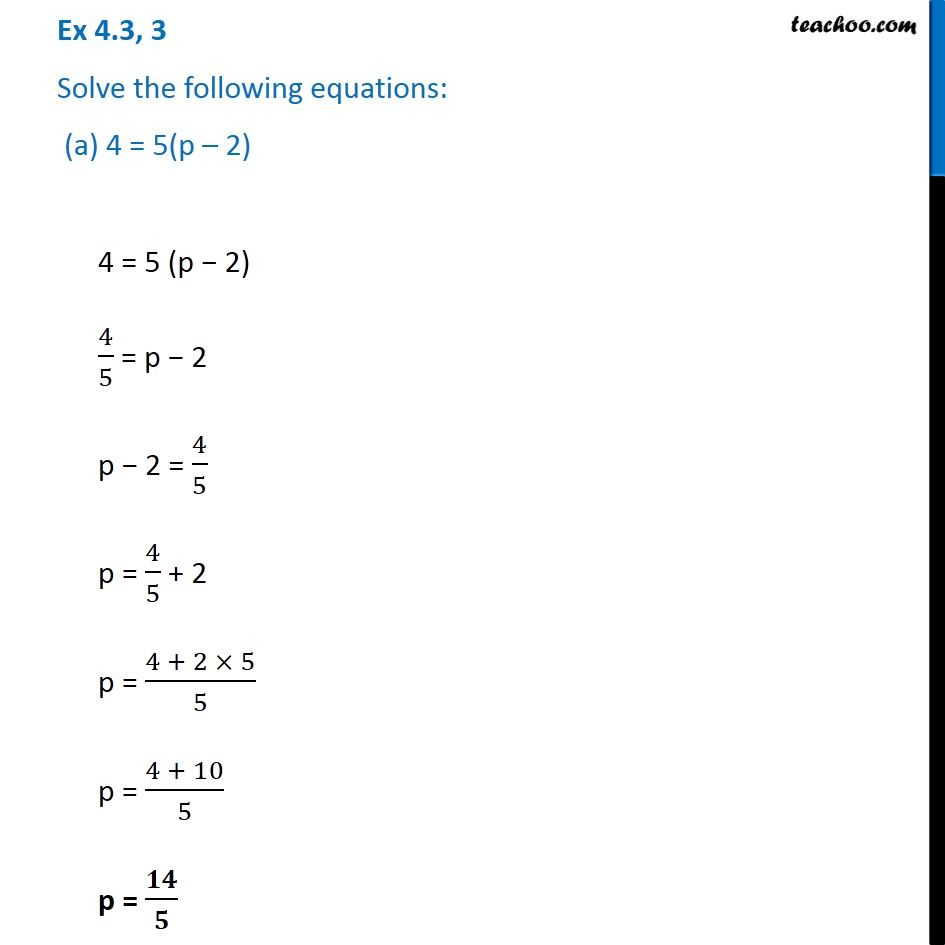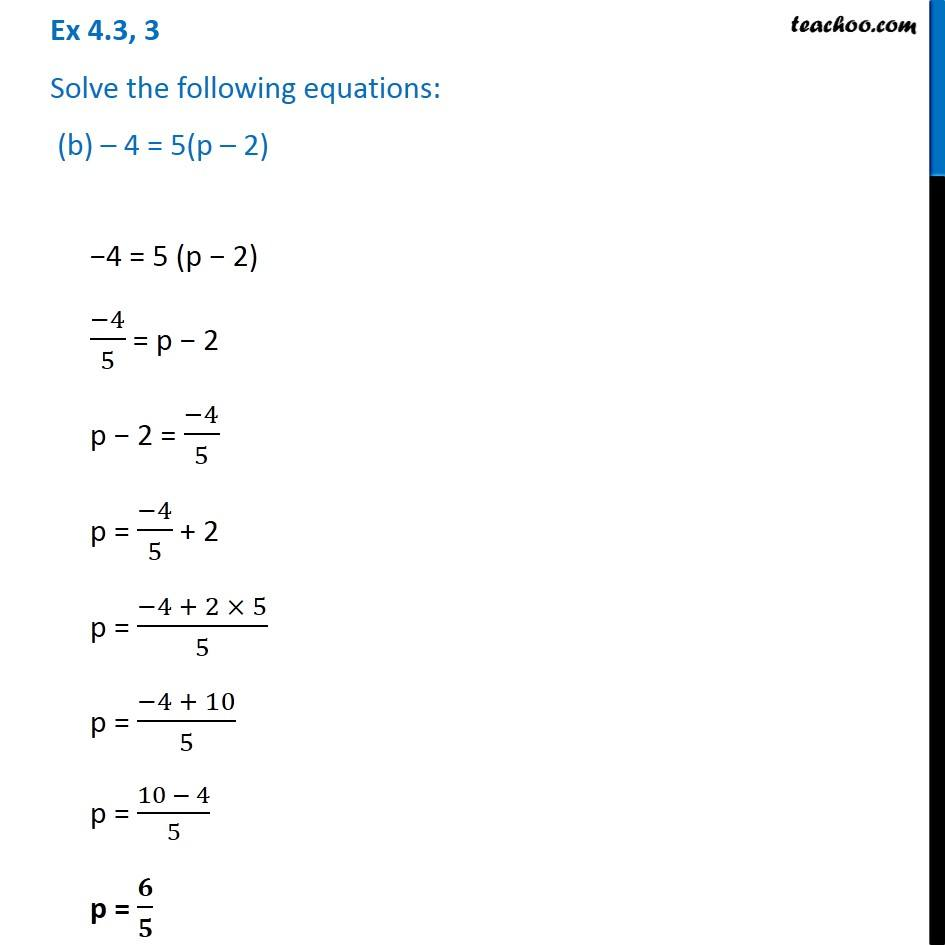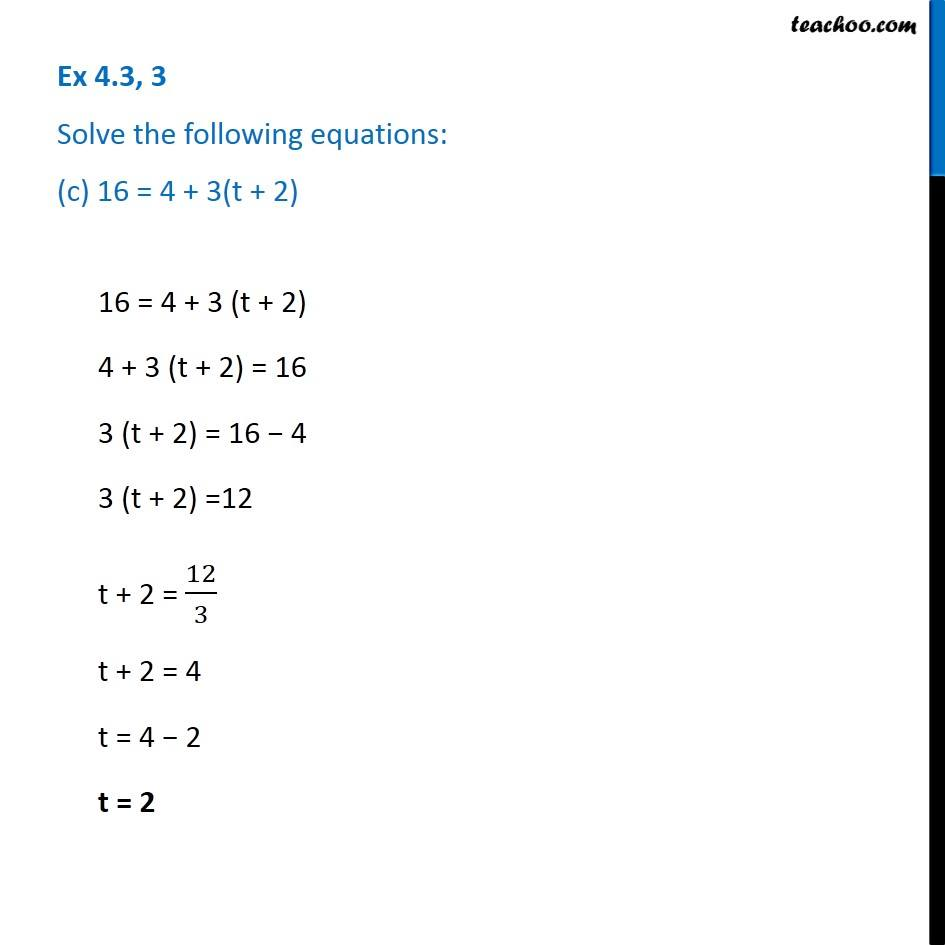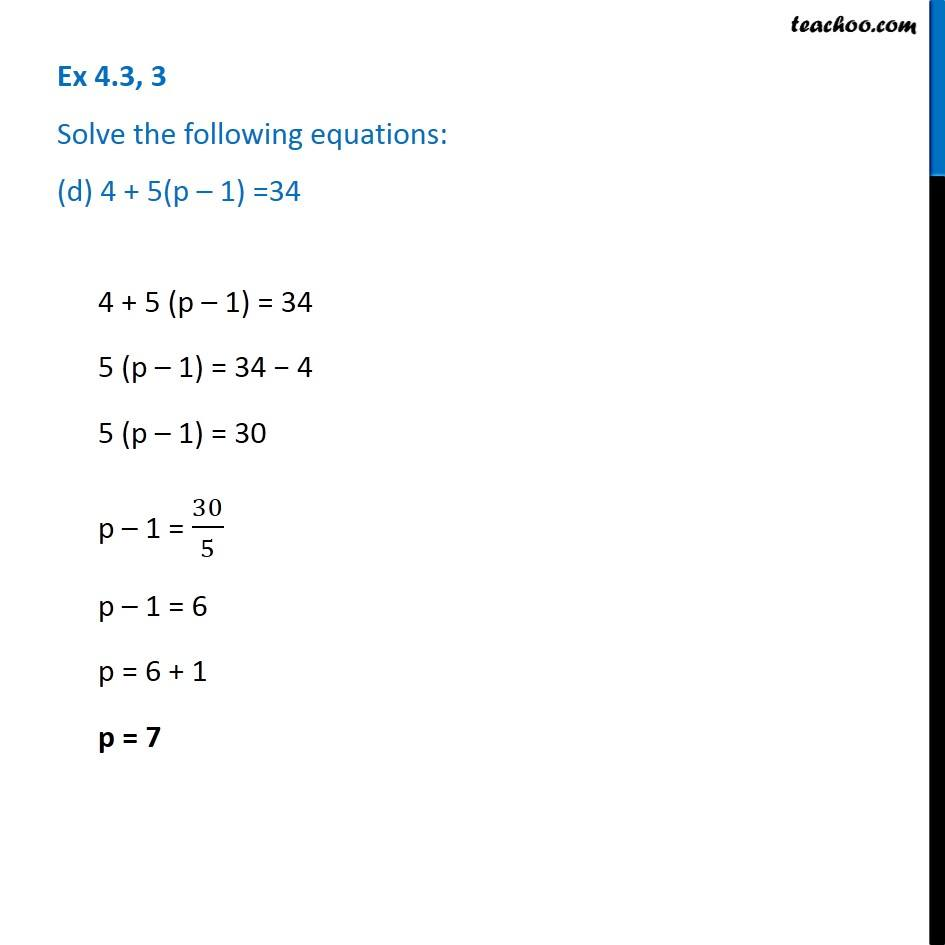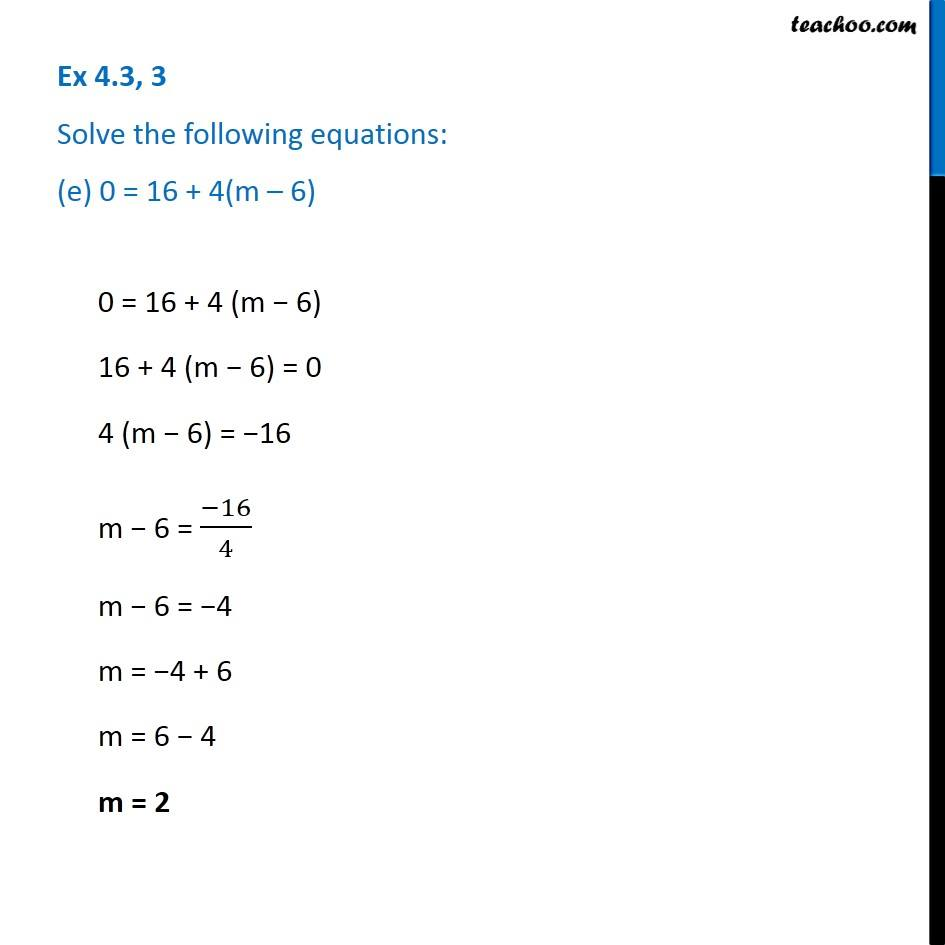Subscribe to our Youtube Channel - https://you.tube/teachoo

1. Chapter 4 Class 7 Simple Equations
2. Serial order wise
3. Ex 4.3

Transcript

Ex 4.3, 3 Solve the following equations: (a) 4 = 5(p – 2)4 = 5 (p − 2) 4/5 = p − 2 p − 2 = 4/5 p = 4/5 + 2 p = (4 + 2 × 5)/5 p = (4 + 10)/5 p = 𝟏𝟒/𝟓 Ex 4.3, 3 Solve the following equations: (b) – 4 = 5(p – 2)−4 = 5 (p − 2) (−4)/5 = p − 2 p − 2 = (−4)/5 p = (−4)/5 + 2 p = (−4 + 2 × 5)/5 p = (−4 + 10)/5 p = (10 − 4)/5 p = 𝟔/𝟓 Ex 4.3, 3 Solve the following equations: (c) 16 = 4 + 3(t + 2)16 = 4 + 3 (t + 2) 4 + 3 (t + 2) = 16 3 (t + 2) = 16 − 4 3 (t + 2) =12 t + 2 = 12/3 t + 2 = 4 t = 4 − 2 t = 2 Ex 4.3, 3 Solve the following equations: (d) 4 + 5(p – 1) =344 + 5 (p – 1) = 34 5 (p – 1) = 34 − 4 5 (p – 1) = 30 p – 1 = 30/5 p – 1 = 6 p = 6 + 1 p = 7 Ex 4.3, 3 Solve the following equations: (e) 0 = 16 + 4(m – 6)0 = 16 + 4 (m − 6) 16 + 4 (m − 6) = 0 4 (m − 6) = −16 m − 6 = (−16)/4 m − 6 = −4 m = −4 + 6 m = 6 − 4 m = 2

Ex 4.3